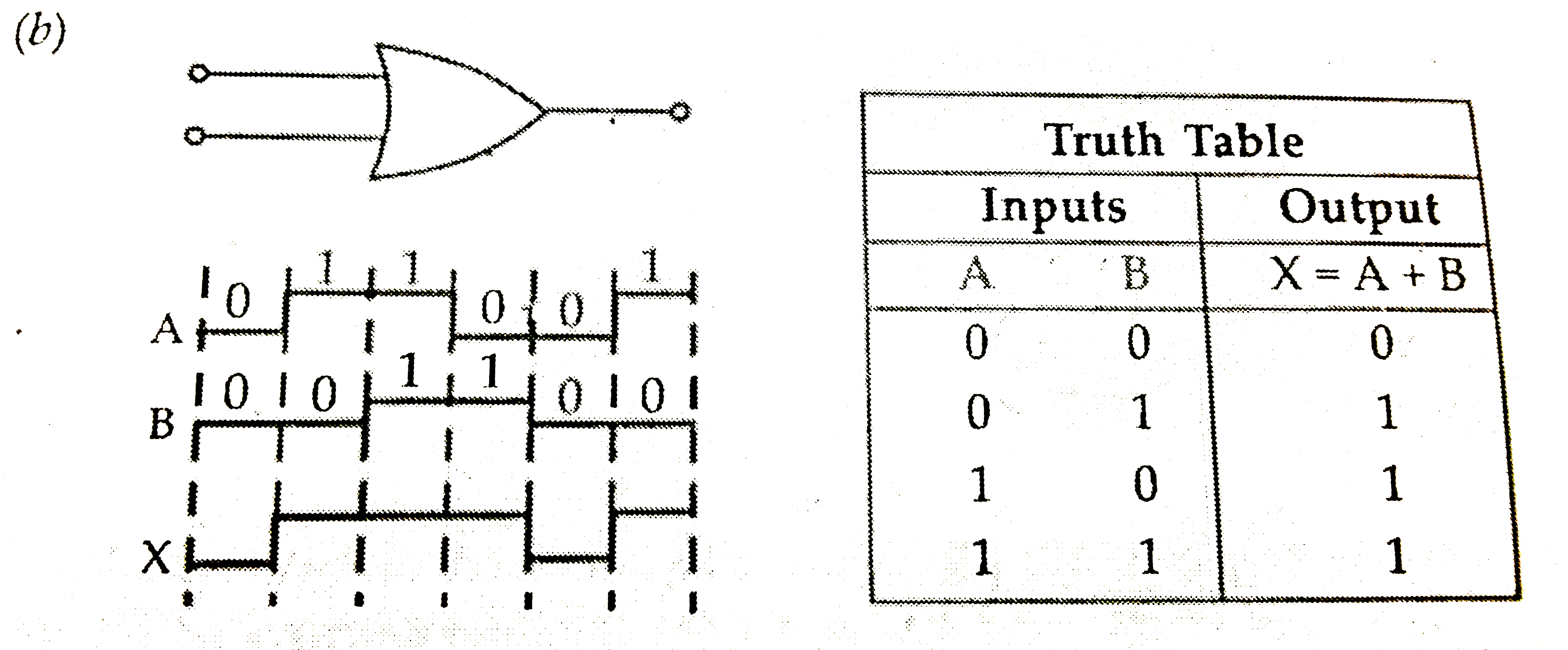# (a) Draw the circuit diagram for studying the characteristics of a transistor in common emitter configuration. Explain briefly and show how input and

54 views
in Physics
closed
(a) Draw the circuit diagram for studying the characteristics of a transistor in common
emitter configuration. Explain briefly and show how input and output characteristics
are drawn.
(b) The figure shows input weve forms A and B to a logic gate . Draw the output waveform
for an OR gate . Write the truth table for this logic gate and draw the output waveform
for an OR gate . Write truth table for this logic gate and draw its logic symbol.

by (87.7k points)
selected

(a) See Q . 30 (a) , Set-I Outside Delhi -2010.
(b).